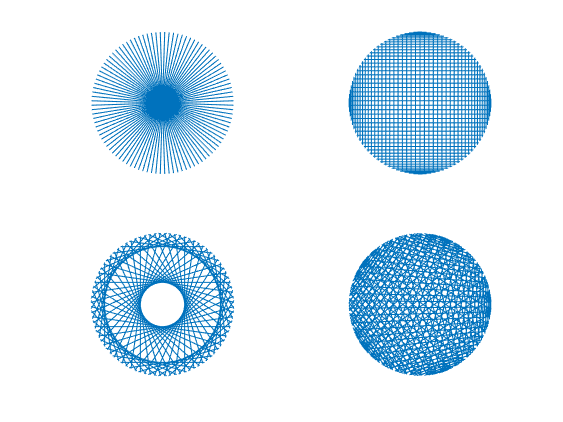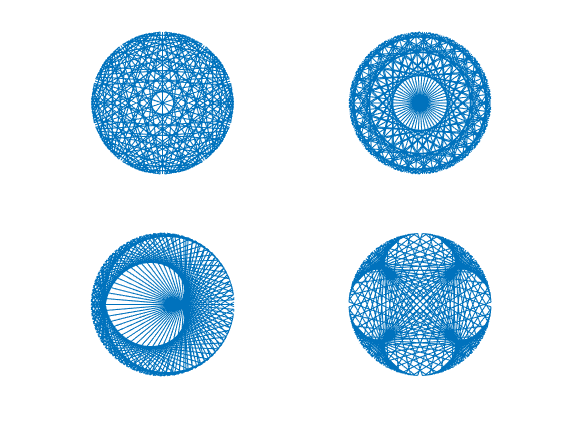This is a crosspost from   Cleve’s Corner: Cleve Moler on Mathematics and Computing Cleve Moler is the author of the first MATLAB, one of the founders of MathWorks, and is currently Chief Mathematician at the company. He writes here about MATLAB, scientific computing and interesting mathematics.. See the original post here.

# modfun, A Short Program Produces Impressive Graphics

This nifty graphics gem started with a contribution by Paul Villain to the MATLAB 2022 Mini Hack, currently taking place on MATLAB Central.

### Contents

#### modfun

Villain's contribution is 102 mod 500 . My rewrite is modfun. Villain's 102 and 500 become the parameters m and n.

```  modfun(m,n) connects n points, z(j), equally spaced
around the complex unit circle, by n+1 straight lines.
The j-th line connects z(j+1) to z(mod(j*m,n)+1).```

#### code

The basic code uses complex arithmetic and is only eight lines long. When the graphics is done with line instead of plot, it is not necessary to use hold on.

```  function modfun(m,n)
init_fig
z = exp(2i*pi*(0:n)/n);
for j = 0:n
zj = [z(j+1),z(mod(j*m,n)+1)];
line(real(zj),imag(zj))
end
end```

The initialization makes line possible.

```  function init_fig
axis([-1 1 -1 1])
axis square
axis off
end```

#### Animation

This animation of modfun(105,200) has one frame for every five lines.#### Gallery

A sample.#### Quiz

Match these calls to modfun to the plots in the Gallery.

```  modfun(88,179)
modfun(89,220)
modfun(99,200)
modfun(101,200)
modfun(111,200)
modfun(113,188)
modfun(126,188)
modfun(126,200)```

#### Software

An interactive modfun.

Get the MATLAB code

Published with MATLAB® R2022a